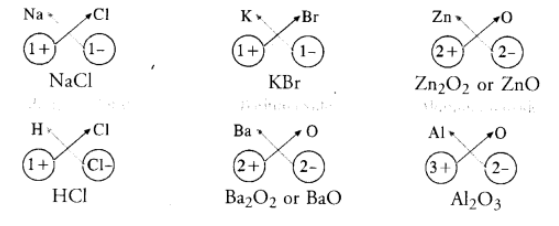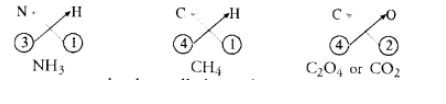# What are ionic and molecular compounds ?`
Question:

What are ionic and molecular compounds ? Give examples.

Solution:

Simple ionic compounds are of binary nature. It means that both the positive and negative ions have one atom only. The symbols of these ions are written side by side with their valencies at their bottom. A common factor if any, is removed to get a simple ratio of the valencies of the combining atoms. The crisscross method is then applied to arrive at the final chemical formula of the compound. Let us write the formulae of a few simple ionic compounds. For example,The molecular compounds also called covalent compounds. The compounds listed above are heteroatomic in nature. It means that different elements participate in these compounds. They may be homoatomic also which means that these are formed from the atoms of the same element. For example, hydrogen molecule $\left(\mathrm{H}_{2}\right)$, chlorine molecule $\left(\mathrm{Cl}_{2}\right)$, oxygen molecule $\left(\mathrm{O}_{2}\right)$, nitrogen molecule $\left(\mathrm{N}_{2}\right)$, etc.